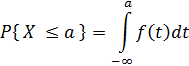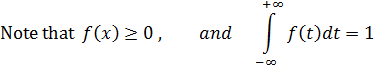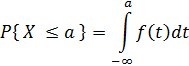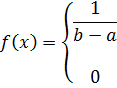Basic Statistics Definitions

1. Probability density function

1. Discrete: If X is a discrete random variable with possible values x1, x2, x3, ..., xn, and f (xi ) denotes P ( X = xi ),  the probability that the

value of X = xi , then   f  is called the (discrete) probability density function (pdf), or simply the density function for X .

Note that, for all x ,   f (xi ) ≥ 0 and i   f (xi) = 1 , where the elements are summed over all i .

2. Continuous: If X is a continous random variable, then the (continuous) probability density function is defined as that function f  such that when integrated as below, gives the probabaility that  X  < a , for some constant a,3.2. Cumulative density function

1. Discrete:

2. Continuous:3. Uniform distribution function

1. Discrete: A uniform probability space is a finite space in which all the outcomes have the same probability. That is, if X is a discrete random variable with event space {x1, x2, x3, ..., xn}, and f (xi ) denotes P ( X = xi ),  then the probability that the value of X is xi , is simply  1/n .That is,  for all i, 1 ≤ in ,  f (xi ) =  1/n
2. Continuous: Let X  be a continous random variable and X ∈ [a,b]. Then the (continuous) uniform probability density function f  is defined byfor a  ≤  x < b otherwise
4. Binomial distribution function

5. Normal or Gaussian Distribution

6. Convolution

1. Discrete:

2. Continuous: